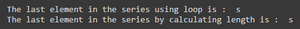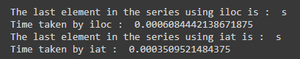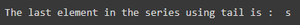# How to access the last element in a Pandas series?

• Last Updated : 26 Nov, 2020

Prerequisite: Pandas

Pandas series is useful in handling various analytical operations independently or as being a part of pandas data frame. So it is important for us to know how various operations are performed in pandas series. The following article discusses various ways in which last element of a pandas series can be retrieved.

Method 1: Naive approach

There are two naive approaches for accessing the last element:

• Iterate through the entire series till we reach the end.
• Find the length of the series. The last element would be length-1 (as indexing starts from 0).

Program:

## Python3

 `# importing the pandas library ` `import` `pandas as pd ` ` `  `# initializing the series ` `ser ``=` `pd.Series([``'g'``, ``'e'``, ``'e'``, ``'k'``, ``'s'``]) ` ` `  `# iterating the series until the iterator reaches the end of the series ` `for` `i ``in` `range``(``0``, ser.size): ` `    ``if` `i ``=``=` `ser.size``-``1``: ` `        ``# printing the last element i.e, size of the series-1 ` `        ``print``(``"The last element in the series using loop is : "``, ser[i]) ` ` `  `# calculating the length of the series ` `len` `=` `ser.size ` ` `  `# printing the last element i.e len-1 as indexing starts from 0 ` `print``(``"The last element in the series by calculating length is : "``, ser[``len``-``1``]) `

Output:Method 2: Using .iloc or .iat

Pandas iloc is used to retrieve data by specifying its integer index. In python negative index starts from end therefore we can access the last element by specifying index to -1 instead of length-1 which will yield the same result.

Pandas iat is used to access data of a passed location. iat is comparatively faster than iloc.  Also note that ser[-1] will not print the last element of series, as series supports positive indexes only. However, we can use negative indexing in iloc and iat.

Program:

## Python3

 `# importing the pandas library and time ` `import` `pandas as pd ` `import` `time ` `# initializing the series ` `ser ``=` `pd.Series([``'g'``, ``'e'``, ``'e'``, ``'k'``, ``'s'``]) ` ` `  `start ``=` `time.time() ` `print``(``"The last element in the series using iloc is : "``, ser.iloc[``-``1``]) ` `end ``=` `time.time() ` ` `  `print``(``"Time taken by iloc : "``, end``-``start) ` ` `  `start ``=` `time.time() ` `print``(``"The last element in the series using iat is : "``, ser.iat[``-``1``]) ` `end ``=` `time.time() ` ` `  `print``(``"Time taken by iat : "``, end``-``start) `

Output:Method 3: Using tail(1).item()

tail(n) is used to access bottom n rows from a series or a data frame and item() returns the element of the given series object as scalar.

Program:

## Python3

 `# importing the pandas library ` `import` `pandas as pd ` `# initializing the series ` `ser ``=` `pd.Series([``'g'``, ``'e'``, ``'e'``, ``'k'``, ``'s'``]) ` ` `  `# printing the last element using tail ` `print``(``"The last element in the series using tail is : "``, ser.tail(``1``).item()) `

Output:My Personal Notes arrow_drop_up
Recommended Articles
Page :